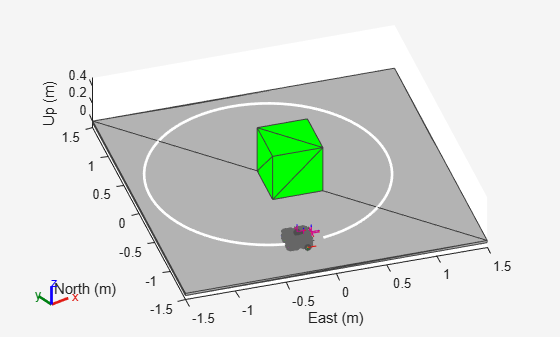Gather latest reading from robot sensor

Since R2022a

## Syntax

``[isUpdated,t,sensorReadings] = read(sensor)``

## Description

example

````[isUpdated,t,sensorReadings] = read(sensor)` gathers the simulated sensor output sensor readings from the latest update of the robot platform associated with the specified sensor `sensor`. The function returns an indicator `isUpdated` of whether the reading was updated at the simulation step in the scenario with timestamp `t`.```

## Examples

collapse all

Create a robot scenario.

`scenario = robotScenario(UpdateRate=100,StopTime=1);`

Add the ground plane and a box as meshes.

```addMesh(scenario,"Plane",Size=[3 3],Color=[0.7 0.7 0.7]); addMesh(scenario,"Box",Size=[0.5 0.5 0.5],Position=[0 0 0.25], ... Color=[0 1 0])```

Create a waypoint trajectory for the robot platform using an ENU reference frame.

```waypoint = [0 -1 0; 1 0 0; -1 1 0; 0 -1 0]; toa = linspace(0,1,length(waypoint)); traj = waypointTrajectory("Waypoints",waypoint, ... "TimeOfArrival",toa, ... "ReferenceFrame","ENU");```

Create a `rigidBodyTree` object of the TurtleBot 3 Waffle Pi robot with `loadrobot`.

`robotRBT = loadrobot("robotisTurtleBot3WafflePi");`

Create a robot platform with trajectory.

```platform = robotPlatform("TurtleBot",scenario, ... BaseTrajectory=traj);```

Set up platform mesh with the `rigidBodyTree` object.

`updateMesh(platform,"RigidBodyTree",Object=robotRBT)`

Create an INS sensor object and attach the sensor to the platform.

```ins = robotSensor("INS",platform,insSensor("RollAccuracy",0), ... UpdateRate=scenario.UpdateRate);```

Visualize the scenario.

```[ax,plotFrames] = show3D(scenario); axis equal hold on```

In a loop, step through the trajectory to output the position, orientation, velocity, acceleration, and angular velocity.

```count = 1; while ~isDone(traj) [Position(count,:),Orientation(count,:),Velocity(count,:), ... Acceleration(count,:),AngularVelocity(count,:)] = traj(); count = count+1; end```

Create a line plot for the trajectory. First create the plot with `plot3`, then manually modify the data source properties of the plot. This improves the performance of the plotting.

```trajPlot = plot3(nan,nan,nan,"Color",[1 1 1],"LineWidth",2); trajPlot.XDataSource = "Position(:,1)"; trajPlot.YDataSource = "Position(:,2)"; trajPlot.ZDataSource = "Position(:,3)";```

Set up the simulation. Then, iterate through the positions and show the scene each time the INS sensor updates. Advance the scene, move the robot platform, and update the sensors.

```setup(scenario) for idx = 1:count-1 % Read sensor readings. [isUpdated,insTimestamp(idx,1),sensorReadings(idx)] = read(ins); if isUpdated % Use fast update to move platform visualization frames. show3D(scenario,FastUpdate=true,Parent=ax); % Refresh all plot data and visualize. refreshdata drawnow limitrate end % Advance scenario simulation time. advance(scenario); % Update all sensors in the scene. updateSensors(scenario) end hold off```## Input Arguments

collapse all

Robot sensor added to platform in scenario, specified as a `robotSensor` object.

## Output Arguments

collapse all

Sensor reading update indicator, returned as a logical `0` (`false`) or `1` (`true`). If the sensor reading updated at the current simulation step, the function returns this argument as `true`.

Data Types: `logical`

Timestamp of the generated sensor reading, returned as a scalar in seconds.

Data Types: `double`

Simulated sensor readings, which depends on the type of sensor specified in the `sensor` input argument. See the Usage syntax for the appropriate `gpsSensor`, `insSensor`, or `robotLidarPointCloudGenerator` System object™.

## Version History

Introduced in R2022a